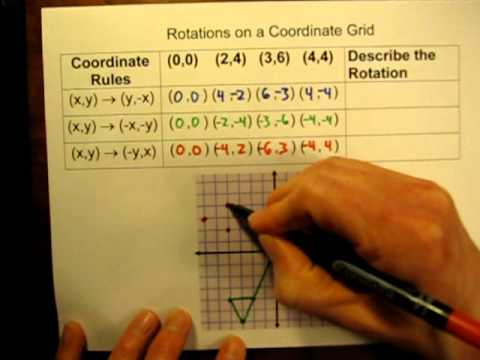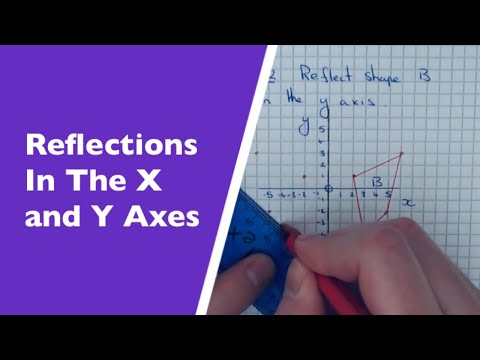## Transformation

Subject: Optional Maths

#### Overview

This note gives the information of congruent transformation and reflection using coordinates.
##### Transformation

Congruent Transformation

Congruent transformation changes in position but no change in the size of an object is called congruent transformation .It is also called isometric transformation .Here, Reflection and Rotation are the examples of congruent transformation.

ReflectIon

A transformation in which a geometric figure is reflected across a line , creating a mirror image . That line is called the axis of a reflection .

Let's see the object given below and try jot down conclusion from the figure .

FIGURE

Conclusion

1. The image lies on the opposite side of the object with respect to the mirror (axis of reflection )
2. The object distance is the distance between the mirror to the object is equal to the image distance is the distance between the mirror to the image .
3. Object size and image size are equal .

A rule which shifts an object is the reflection to the objects of same form each being at an equal distance from a fixed line .And this fixed line is known as mirror or axis of reflection .Reflection using coordinates

As we know, the coordinate system has been well used .In coordinate plane, we can reflect the objects. Here, the coordinates of geometrical figures formed after the reflection about x-axis and y-axis . X-axis and Y-axis are known as the axis of reflection .

x-axis as the axis of reflection

Look at the following figure, and learn to find the coordinates of the image of a point in different quadrants when x-axis is the axis of reflection .

From the above figure, when a geometrical figure is reflected about x-axis as the axis of reflection, the x-coordinate of the image remains the same and the sign of y-coordinate of the image is changed .

FIGURE

y-axis as the axis of reflection: Look the following figure and learn the coordinate of the image of a point in different quadrants when y-axis is the axis of reflection .FIGURE

Look the following figure and learn to find the coordinates of the image of a point in different quadrants when y-axis is the axis of reflection .

FIGURE

Rotation

A transformation in which a plane figure turns around a fixed center point . In other words , one point on the plane the center of rotation , is fixed and everything else on the plane rotates about that point by a given angle .

Study the following figure and find out the idea of rotation of a point.

FIGURE

In the same way, each vertex of a geometrical figure are rotated through a certain angle in a certain direction about a given center of rotation , this figure is rotated through the same angle in the same direction about the same centre of rotation .To rotate this geometrical figure, these three conditions are required ;

1. Centre of rotation 2.Angle of rotation 3. Direction of rotation

A figure can be rotated in two directions.

1. Anti-clockwise direction ( positive direction )

Here, a figure rotates in opposite hand of a clock in an anti-clockwise direction.

1. Clockwise direction ( Negative direction )

Here, In the clockwise direction, we rotate a figure in the same direction of the rotation of the hand of a clock.

Now learn how to draw the image of a figure when it is rotated through the given angle in the given direction about a given centre of rotation.

Rotation through 900 in anti-clockwise direction FIGURE

1.The dotted line of each vertex of figure should join to the centre of rotation

2.Draw 900 at o in each dotted lines with the help of a protractor in an anti-clockwise direction .

3.Cut off 0A’ = 0A, 0B’ = 0B and 0C’ = 0C.

4.Now, join A’, B’, and C’ .

Rotation through 900 in clockwise direction

FIGURE

Rotation of geometrical figure using coordinates

Look the following figure and learn to find out the coordinates of the image of a point when it is rotated through 900 and 1800 in anti-clockwise and clockwise directions about the centre of rotation at the origin .

Rotation through 900 in anti-clockwise about origin

FIGURE

Rotation through 900 in clockwise about origin

FIGURE

In clockwise, a point is rotated through 90 in a direction about the origin as the centre of rotation, x and y-coordinates are exchanged by making the sign of y-coordinate just opposite.

Rotation through 1800 in anti- clockwise and clockwise about origin

Here a point rotated through 1800 about the origin , then the coordinates of the image are the same in both directions. Learn the following figure .

FIGURE

In anti-clockwise, a point is rotated through 900 or in a clockwise direction about the origin as the centre of rotation ,x and y-coordinates the image remains the same just by changing their signs.

##### Things to remember
• Congruent transformation  change in  position but no change in size of  an objects .
• Fixed line is called  the mirror or axis of reflection .
• Congruent transformation also known as  isometric transformation.
• It includes every relationship which established among the people.
• There can be more than one community in a society. Community smaller than society.
• It is a network of social relationships which cannot see or touched.
• common interests and common objectives are not necessary for society.
##### Videos for Transformation##### Coordinate Rules for Rotations on a Graph.##### How To Reflect A Shape In The X-Axis Or Y-Axis On A Coordinate Grid.##### Rotations on the Coordinate Grid

Solution:

According to the question,

A (3, -3) → A ' (-3, -3)

B( 4, 2) → B ' (-4, 3)

C(1, 3) → C ' (-1, 3)

So, the coordinates of the vertices of image ΔA'B'C' are A' (-3, -3), B(-4, 3) and C' (-1, 3)

Solution:

According to the given information,

A(-3, 4) → A' (-3, -4)

B(3,2) → B' (3, -2)

C(5,5) → C' (5, -5)

So, the coordinates of the vertices of image ΔA'B'C' are A' (-3, -4), B' (3, -2) and  c' (5, -5).

Solution

Here, A(2,4) translated by  ($\frac{-5}{2}$) and then ( 2-5, 4 +2) = (-3,6) , i.e C is obtained. The distance from B to D, N to M and C are parallel as well as equal. So, M maps N and D maps B.

Solution:

According to the question,

A (-2, 3) → A ' (-2, -3)

B( 1, 2) → B ' (1, -2)

C(3, 3) → C ' (3, -3)

So, the coordinates of the vertices of image ΔA'B'C' are A' (-2, -3), B(1, -2) and C' (3, -3)

Solution:

According to the question,

A (2, -3) → A ' (-2,-3)

B( 2, 1) → B ' (-2, 1)

C(3, 2) → C ' (-3, 2)

So, the coordinates of the vertices of image ΔA'B'C' are A' (-2, -3), B(-2, 3) and C' (-3, 2)

Solution:

According to the question,

A(-1, -2) → A ' (-1, 2)

B( 3, 2) → B ' (3, -2)

C(1, 2) → C ' (1, -2)

So, the coordinates of the vertices of image ΔA'B'C' are A' (-1, -2), B(3, -2) and C' (1, -2)

Solution:

Join each of the vertex to the centre of rotation.

Draw angle of 90O at O with OC, OA  and OB in an anticlockwise direction.

Cut OA = OA' , OC' = OC'  and OB = OB'

Join  A' B' and  C'

ΔA'B'C'  is image  of ΔABC

Solution

P(x,y) → P' (-y, x)

A(-3, 4) → A(4, 3)

B(4,3) →B(-4, 3)

Solution:

According to the question,

When  ΔABC is rotated through 90º in an  anti-clockwise direction about the origin.

A(3,2)→ A' (-3, 2)

B' (2,1)→B' (-2, 1)

C(2,3) →C' (-2, 3)

So, the coordinates of the vertices of image ΔA'B'C are A (-3, 2), B(-2, 1) and C(-2, 3).

Transformation is the process  of changing the object either in position or in size or in both.

Reflection is a rule which shifts the same object to the object form of  each being  at an equal distance from the fixed line.

The fixed point is known as the centre of rotation.

Reflection and Rotation are the examples of congruent transformation.

In the clockwise direction (Negative direction), we rotate a figure in the same direction of the rotation.